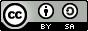# 情感分析¶

## 说明:¶

1. 硬件环境要求： 本文可支持在CPU、GPU下运行
2. Docker镜像支持的CUDA/cuDNN版本： 如果使用了Docker运行Book，请注意：这里所提供的默认镜像的GPU环境为 CUDA 8/cuDNN 5，对于NVIDIA Tesla V100等要求CUDA 9的 GPU，使用该镜像可能会运行失败。
3. 文档和脚本中代码的一致性问题： 请注意：为使本文更加易读易用，我们拆分、调整了train.py的代码并放入本文。本文中代码与train.py的运行结果一致，可直接运行train.py进行验证。

## 模型概览¶

### 文本卷积神经网络简介（CNN）¶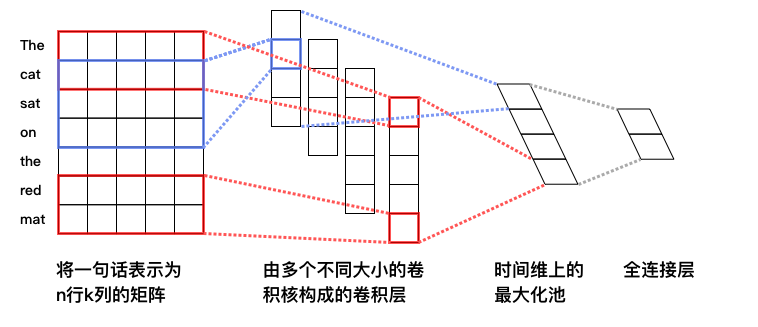### 循环神经网络（RNN）¶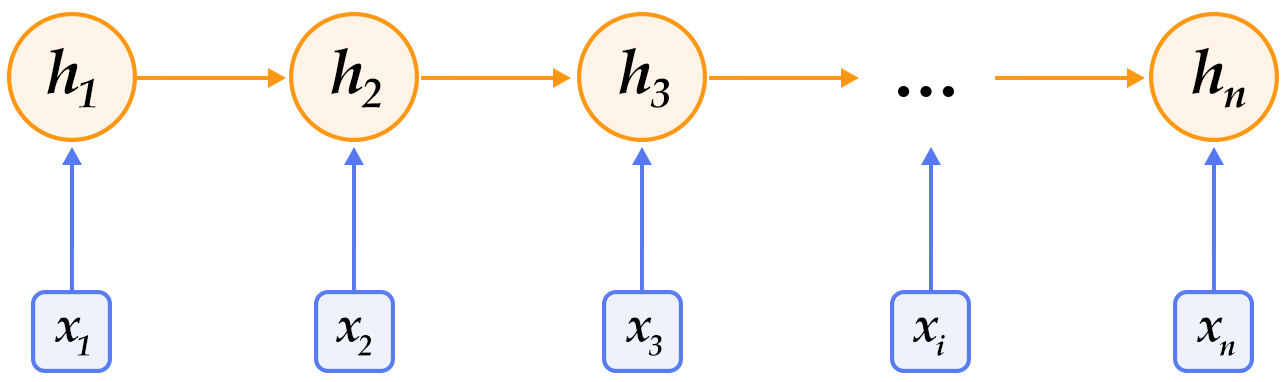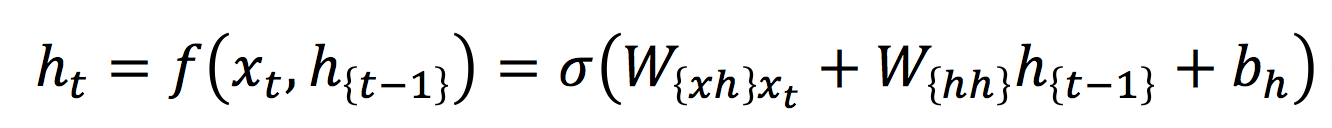### 长短期记忆网络（LSTM）¶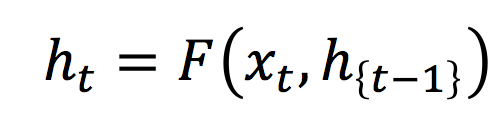$F$由下列公式组合而成：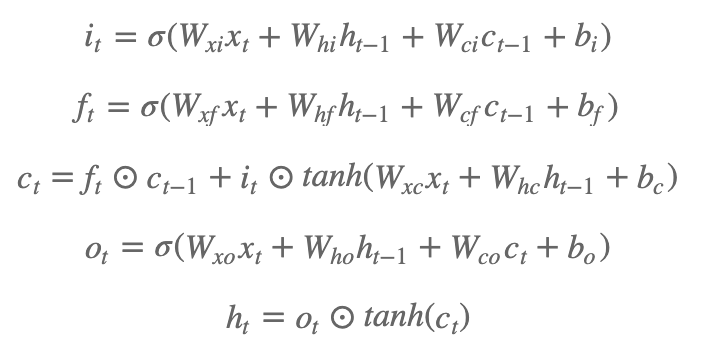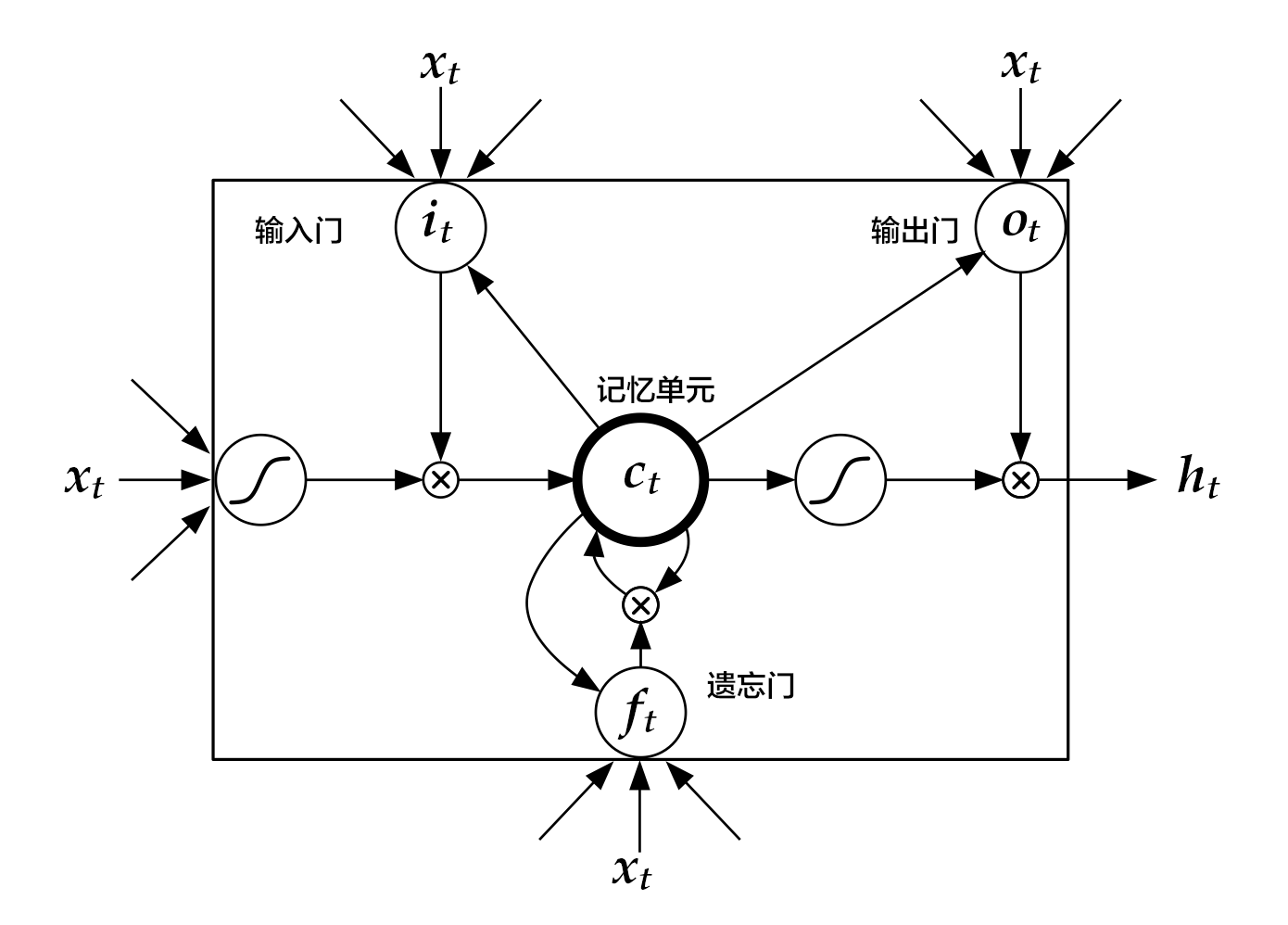LSTM通过给简单的循环神经网络增加记忆及控制门的方式，增强了其处理远距离依赖问题的能力。类似原理的改进还有Gated Recurrent Unit (GRU)，其设计更为简洁一些。这些改进虽然各有不同，但是它们的宏观描述却与简单的循环神经网络一样（如图2所示），即隐状态依据当前输入及前一时刻的隐状态来改变，不断地循环这一过程直至输入处理完毕：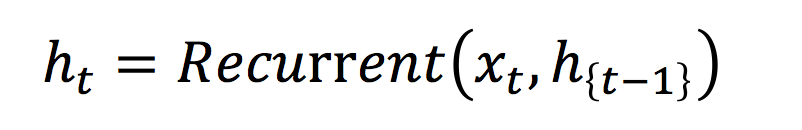### 栈式双向LSTM（Stacked Bidirectional LSTM）¶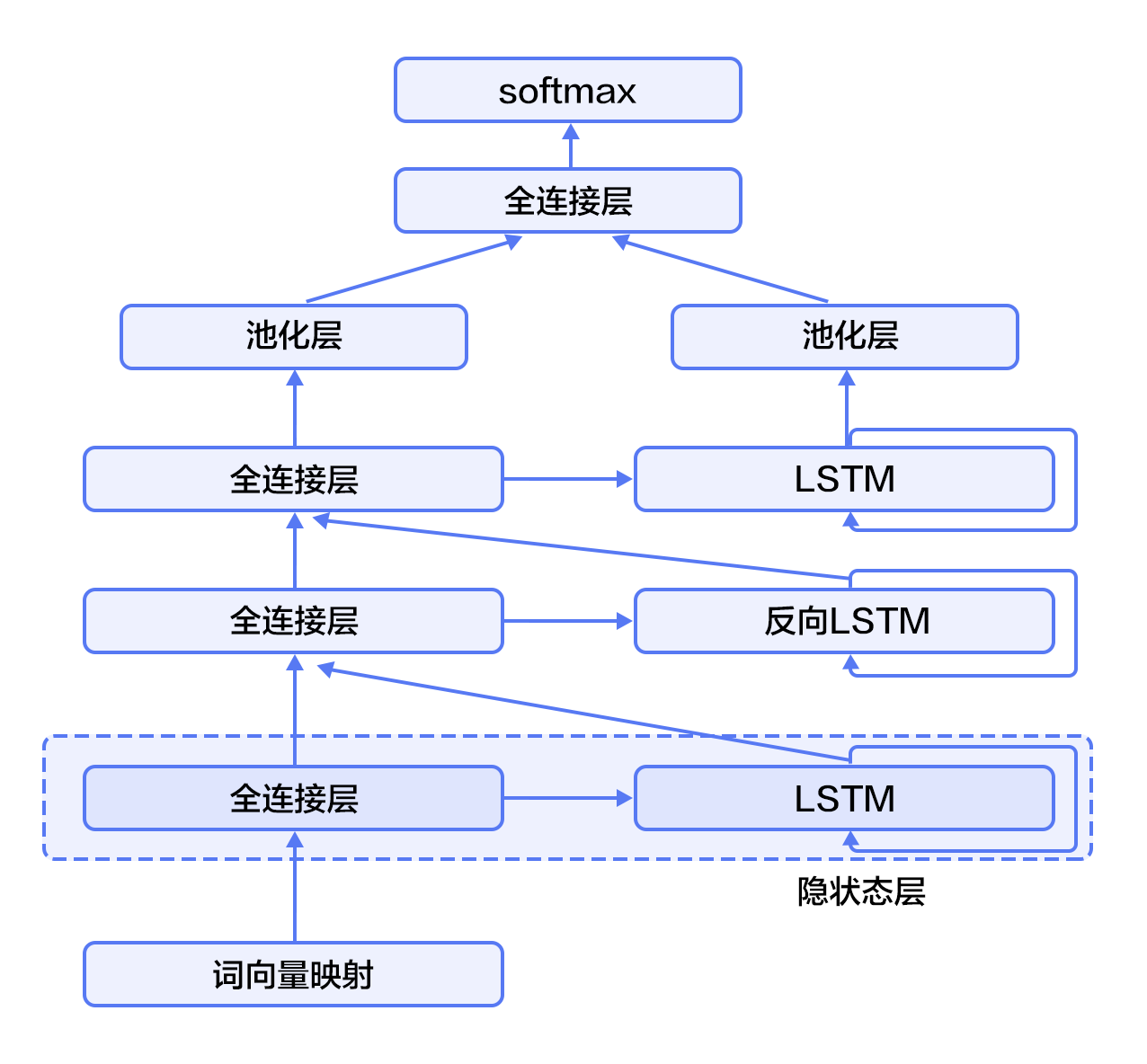## 数据集介绍¶

aclImdb
|- test
|-- neg
|-- pos
|- train
|-- neg
|-- pos


Paddle在dataset/imdb.py中提实现了imdb数据集的自动下载和读取，并提供了读取字典、训练数据、测试数据等API。

## 配置模型¶

from __future__ import print_function
import numpy as np
import sys
import math

CLASS_DIM = 2     #情感分类的类别数
EMB_DIM = 128     #词向量的维度
HID_DIM = 512     #隐藏层的维度
STACKED_NUM = 3   #LSTM双向栈的层数
BATCH_SIZE = 128  #batch的大小


### 文本卷积神经网络¶

#文本卷积神经网络
def convolution_net(data, input_dim, class_dim, emb_dim, hid_dim):
emb = fluid.layers.embedding(
input=data, size=[input_dim, emb_dim], is_sparse=True)
conv_3 = fluid.nets.sequence_conv_pool(
input=emb,
num_filters=hid_dim,
filter_size=3,
act="tanh",
pool_type="sqrt")
conv_4 = fluid.nets.sequence_conv_pool(
input=emb,
num_filters=hid_dim,
filter_size=4,
act="tanh",
pool_type="sqrt")
prediction = fluid.layers.fc(
input=[conv_3, conv_4], size=class_dim, act="softmax")
return prediction


### 栈式双向LSTM¶

#栈式双向LSTM
def stacked_lstm_net(data, input_dim, class_dim, emb_dim, hid_dim, stacked_num):

#计算词向量
emb = fluid.layers.embedding(
input=data, size=[input_dim, emb_dim], is_sparse=True)

#第一层栈
#全连接层
fc1 = fluid.layers.fc(input=emb, size=hid_dim)
#lstm层
lstm1, cell1 = fluid.layers.dynamic_lstm(input=fc1, size=hid_dim)

inputs = [fc1, lstm1]

#其余的所有栈结构
for i in range(2, stacked_num + 1):
fc = fluid.layers.fc(input=inputs, size=hid_dim)
lstm, cell = fluid.layers.dynamic_lstm(
input=fc, size=hid_dim, is_reverse=(i % 2) == 0)
inputs = [fc, lstm]

#池化层
fc_last = fluid.layers.sequence_pool(input=inputs, pool_type='max')
lstm_last = fluid.layers.sequence_pool(input=inputs, pool_type='max')

#全连接层，softmax预测
prediction = fluid.layers.fc(
input=[fc_last, lstm_last], size=class_dim, act='softmax')
return prediction


def inference_program(word_dict):
data = fluid.layers.data(
name="words", shape=, dtype="int64", lod_level=1)

dict_dim = len(word_dict)
net = convolution_net(data, dict_dim, CLASS_DIM, EMB_DIM, HID_DIM)
# net = stacked_lstm_net(data, dict_dim, CLASS_DIM, EMB_DIM, HID_DIM, STACKED_NUM)
return net


def train_program(prediction):
label = fluid.layers.data(name="label", shape=, dtype="int64")
cost = fluid.layers.cross_entropy(input=prediction, label=label)
avg_cost = fluid.layers.mean(cost)
accuracy = fluid.layers.accuracy(input=prediction, label=label)
return [avg_cost, accuracy]   #返回平均cost和准确率acc

#优化函数
def optimizer_func():


## 训练模型¶

### 定义训练环境¶

use_cuda = False  #在cpu上进行训练
place = fluid.CUDAPlace(0) if use_cuda else fluid.CPUPlace()


### 定义数据提供器¶

print("Loading IMDB word dict....")

batch_size=BATCH_SIZE)


word_dict是一个字典序列，是词和label的对应关系，运行下一行可以看到具体内容：

word_dict


### 构造训练器¶

exe = fluid.Executor(place)
prediction = inference_program(word_dict)
[avg_cost, accuracy] = train_program(prediction)#训练程序
sgd_optimizer = optimizer_func()#训练优化函数
sgd_optimizer.minimize(avg_cost)


def train_test(program, reader):
count = 0
feed_var_list = [
program.global_block().var(var_name) for var_name in feed_order
]
feeder_test = fluid.DataFeeder(feed_list=feed_var_list, place=place)
test_exe = fluid.Executor(place)
accumulated = len([avg_cost, accuracy]) * 
avg_cost_np = test_exe.run(
program=program,
feed=feeder_test.feed(test_data),
fetch_list=[avg_cost, accuracy])
accumulated = [
x + x for x in zip(accumulated, avg_cost_np)
]
count += 1
return [x / count for x in accumulated]


### 提供数据并构建主训练循环¶

feed_order用来定义每条产生的数据和fluid.layers.data之间的映射关系。比如，imdb.train产生的第一列的数据对应的是words这个特征。

# Specify the directory path to save the parameters
params_dirname = "understand_sentiment_conv.inference.model"

feed_order = ['words', 'label']
pass_num = 1  #训练循环的轮数

#程序主循环部分
def train_loop(main_program):
#启动上文构建的训练器
exe.run(fluid.default_startup_program())

feed_var_list_loop = [
main_program.global_block().var(var_name) for var_name in feed_order
]
feeder = fluid.DataFeeder(
feed_list=feed_var_list_loop, place=place)

test_program = fluid.default_main_program().clone(for_test=True)

#训练循环
for epoch_id in range(pass_num):
#运行训练器
metrics = exe.run(main_program,
feed=feeder.feed(data),
fetch_list=[avg_cost, accuracy])

#测试结果
print('Step {0}, Test Loss {1:0.2}, Acc {2:0.2}'.format(
step_id, avg_cost_test, acc_test))

print("Step {0}, Epoch {1} Metrics {2}".format(
step_id, epoch_id, list(map(np.array,
metrics))))

if step_id == 30:
if params_dirname is not None:
fluid.io.save_inference_model(params_dirname, ["words"],
prediction, exe)#保存模型
return


### 开始训练¶

train_loop(fluid.default_main_program())


## 应用模型¶

### 构建预测器¶

place = fluid.CUDAPlace(0) if use_cuda else fluid.CPUPlace()
exe = fluid.Executor(place)
inference_scope = fluid.core.Scope()


### 生成测试用输入数据¶

reviews_str = [
'read the book forget the movie', 'this is a great movie', 'this is very bad'
]
reviews = [c.split() for c in reviews_str]

UNK = word_dict['<unk>']
lod = []
for c in reviews:
lod.append([word_dict.get(words, UNK) for words in c])

base_shape = [[len(c) for c in lod]]

tensor_words = fluid.create_lod_tensor(lod, base_shape, place)


## 应用模型并进行预测¶

with fluid.scope_guard(inference_scope):

[inferencer, feed_target_names,

assert feed_target_names == "words"
results = exe.run(inferencer,
feed={feed_target_names: tensor_words},
fetch_list=fetch_targets,
return_numpy=False)
np_data = np.array(results)
for i, r in enumerate(np_data):
print("Predict probability of ", r, " to be positive and ", r,
" to be negative for review \'", reviews_str[i], "\'")


## 参考文献¶

1. Kim Y. Convolutional neural networks for sentence classification[J]. arXiv preprint arXiv:1408.5882, 2014.
2. Kalchbrenner N, Grefenstette E, Blunsom P. A convolutional neural network for modelling sentences[J]. arXiv preprint arXiv:1404.2188, 2014.
3. Yann N. Dauphin, et al. Language Modeling with Gated Convolutional Networks[J] arXiv preprint arXiv:1612.08083, 2016.
4. Siegelmann H T, Sontag E D. On the computational power of neural nets[C]//Proceedings of the fifth annual workshop on Computational learning theory. ACM, 1992: 440-449.
5. Hochreiter S, Schmidhuber J. Long short-term memory[J]. Neural computation, 1997, 9(8): 1735-1780.
6. Bengio Y, Simard P, Frasconi P. Learning long-term dependencies with gradient descent is difficult[J]. IEEE transactions on neural networks, 1994, 5(2): 157-166.
7. Graves A. Generating sequences with recurrent neural networks[J]. arXiv preprint arXiv:1308.0850, 2013.
8. Cho K, Van Merriënboer B, Gulcehre C, et al. Learning phrase representations using RNN encoder-decoder for statistical machine translation[J]. arXiv preprint arXiv:1406.1078, 2014.
9. Zhou J, Xu W. End-to-end learning of semantic role labeling using recurrent neural networks[C]//Proceedings of the Annual Meeting of the Association for Computational Linguistics. 2015.